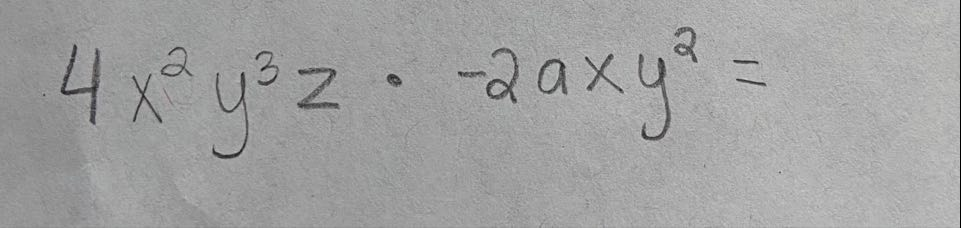# Help Zone

### Student Question

Hello, how to multiply algebraic expressions?

I have these two monomials.Mathematics## Explanations (1)

•Explanation from AlloprofExplanation from Alloprof

This Explanation was submitted by a member of the Alloprof team.

Hi,

First, to multiply two monomials, you must:

1. Multiply the coefficients of the two monomials.
2. Add the exponents affecting identical variables.

In your case, we multiply the coefficients 4 and -2.

(4) ⋅ (−2) = - 8

We add the exponents of the same variables.

a, x2 + 1y3 + 2etz

We only find a and z once, so there is no addition to perform.

The complete process would be as follows.

4x2y3z⋅ − 2axy2 = −2⋅4⋅a⋅x2⋅x⋅y3⋅y2⋅z = −2⋅4⋅a⋅x2⋅x1⋅y3⋅y2⋅z = −8⋅a⋅x2 + 1⋅y3 + 2⋅z = −8⋅a⋅x3⋅y5⋅z

Good luck!

Karen